# Jill Stephenson

•### JST17 12. Section 1a Vector addition and subtraction

Activity

Jill Stephenson

•### JST17 10. Section 2b Stationary points on a cubic curve

Activity

Jill Stephenson

•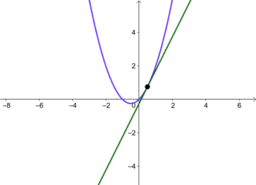### JST17 10. Section 2a Stationary point on a quadratic curve

Activity

Jill Stephenson

•### JST17 10. Section 1c The gradient of a curve using a tangent

Activity

Jill Stephenson

•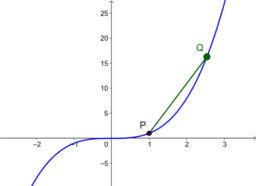### JST17 10. Section 1b Gradient of a chord on a cubic curve

Activity

Jill Stephenson

•### JST17 10. Section 1a Gradient of a chord on a quadratic curve

Activity

Jill Stephenson

•### JST17 5. Section 2d Perpendicular to chord

Activity

Jill Stephenson

•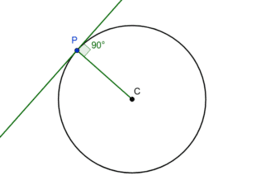### JST17 5. Section 2c Tangent and radius

Activity

Jill Stephenson

•### JST17 5. Section 2b Angle in a semicircle

Activity

Jill Stephenson

•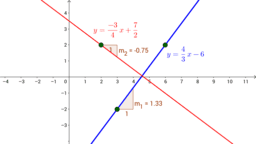### JST17 5. Section 1h Perpendicular lines

Activity

Jill Stephenson

•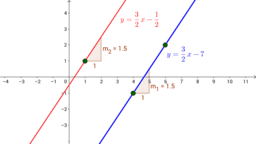### JST17 5. Section 1g Parallel lines

Activity

Jill Stephenson

•### JST17 5. Section 2a Circles

Activity

Jill Stephenson

•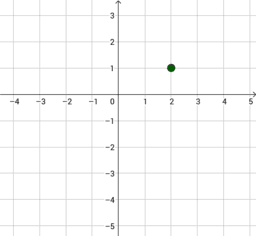### JST17 5. Section 1f Equation of a line from gradient and a point

Activity

Jill Stephenson

•### JST17 5. Section 1e The form ax + by + c = 0

Activity

Jill Stephenson

•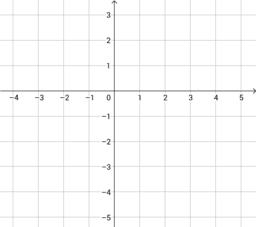### JST17 5. Section 1d The form y = mx + c

Activity

Jill Stephenson

•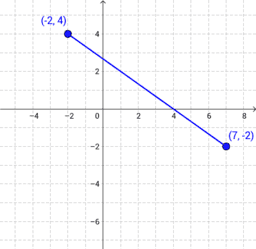### JST17 5. Section 1c The gradient of a line joining two points

Activity

Jill Stephenson

•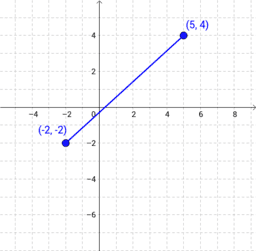### JST17 5. Section 1b The distance between two points

Activity

Jill Stephenson

•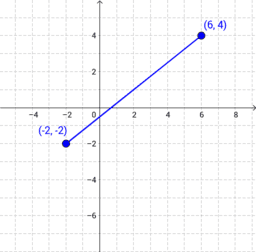### JST17 5. Section 1a The midpoint of two points

Activity

Jill Stephenson

•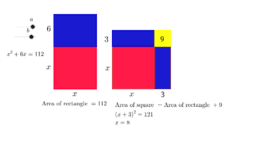### JST17 3. 1b Completing the square and area

Activity

Jill Stephenson

•### JST17 3. 1a Completing the square and graphs

Activity

Jill Stephenson

•### JST17 19. Section 2c The area under a velocity-time graph

Activity

Jill Stephenson

•### JST17 19. Section 2b Describing a graph

Activity

Jill Stephenson

•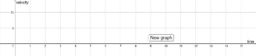### JST17 19. Section 2a The gradient of a velocity-time graph

Activity

Jill Stephenson

•### JST17 19. Section 1 Displacement-time graphs

Activity

Jill Stephenson